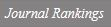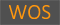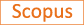View : 350 Download: 0

Evaluation of the convolution sums ∑ak + bl + cm = nσ(k)σ(l)σ(m) with lcm(a,b,c)≤6

Title
Evaluation of the convolution sums ∑ak + bl + cm = nσ(k)σ(l)σ(m) with lcm(a,b,c)≤6
Authors
Park Y.K.
Ewha Authors
SCOPUS Author ID
박윤경Issue Date
2016
Journal Title
Journal of Number Theory
ISSN
0022-314XCitation
Journal of Number Theory vol. 168, pp. 257 - 275
Keywords
Convolution sumQuasimodular formThe number of representation by quadratic forms
Publisher
Academic Press Inc.
Indexed
SCI; SCIE; SCOPUSDocument Type
Article
Abstract
The generating functions of divisor functions are quasimodular forms and their products belong to a space of quasimodular forms of higher weight. In this work, we evaluate the convolution sums ∑ak+bl+cm=nσ(k)σ(l)σ(m) for all positive integers a,b,c,n with lcm(a,b,c)≤6. The evaluation of this convolution sum in the case (a,b,c)=(1,1,1) is due to Lahiri  and in the cases (a,b,c)=(1,1,2),(1,2,2) and (1,2,4) to Alaca, Uygul and Williams . As an application, the known formulas for the number of representations of a positive integer n by each of the quadratic forms∑j=012xj 2 and ∑j=16(x2j−1 2+x2j−1x2j+x2j 2) are reproved using new identities proved in this paper. © 2016 Elsevier Inc.
DOI
10.1016/j.jnt.2016.04.025
Appears in Collections:
연구기관 > 수리과학연구소 > Journal papers
Files in This Item:
There are no files associated with this item.
Export
RIS (EndNote)
XLS (Excel)
XMLItems in DSpace are protected by copyright, with all rights reserved, unless otherwise indicated.